# Visualize Data Structures in VSCode

## September 17, 2020

VSCode Debug Visualizer is a VSCode extension that allows you to visualize data structures in your editor. This can be useful for visualizing watched values during debugging. I've found the extension can be helpful to visualize plots, tables, arrays, histograms and trees.

Once the extension is installed, open some relevant scripts then navigate to the command palette and go to `Debug Visualizer: New View`. This view allows you to enter expressions that are visualized while stepping through your code. It's very similar to VS Code's watch view, with the core difference being that results are visualized rather than displayed in a text format.

If you have a selection of text you want to visualize, `Debug Visualizer: Use Selection as Expression` can evaluate the current text you have selected in the last debug visualizer window you opened.

## Official examples

The JavaScript examples for VSCode Debug Visualizer are included in the main repository. To get started with them, check you have `yarn` and `node` installed, then:

• Clone the repository locally. Navigate to the `demos/js` directory once that's done.
• Run `yarn` to install the dependencies. I needed to manually run `yarn add @hediet/debug-visualizer-data-extraction` to get everything working.
• Open the repo up in VSCode and select an example. e.g `demo_doubly-linked-list.ts`

Add a breakpoint / `debugger` statement somewhere in the source (e.g line 50).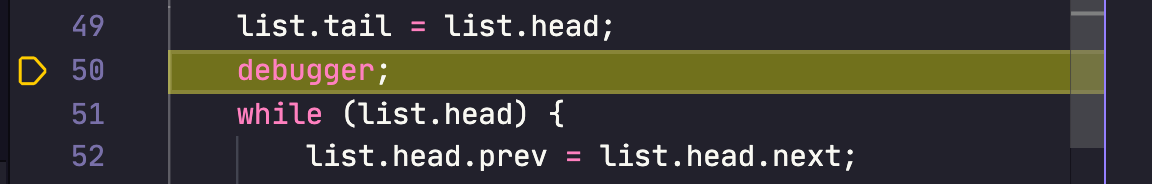Go to Run > Start Debugging and select an environment (e.g `Node.js (preview)`).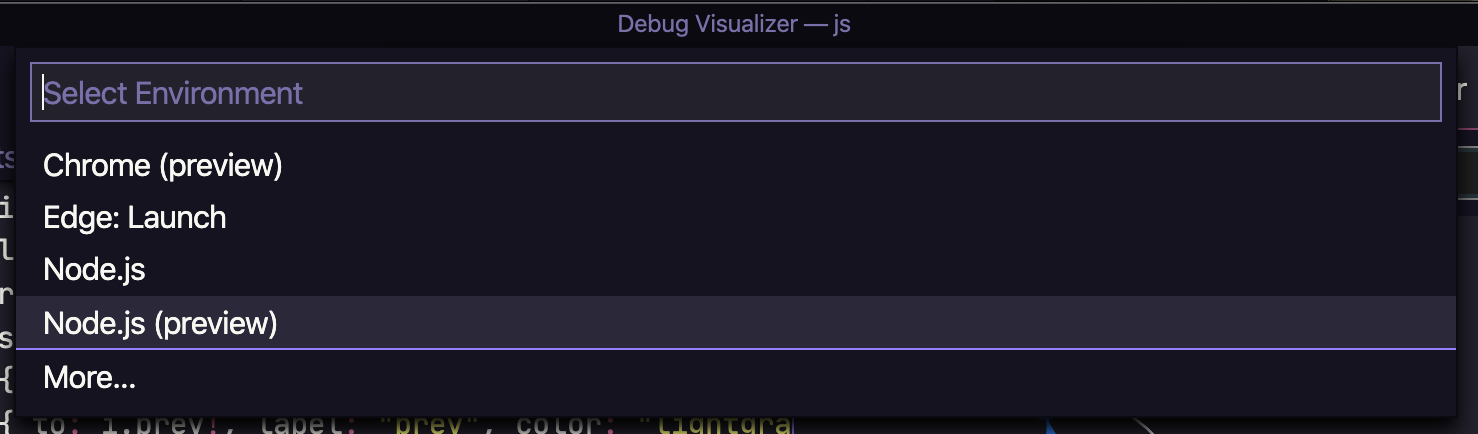From the command-palette, select `Debug Visualizer: New View`.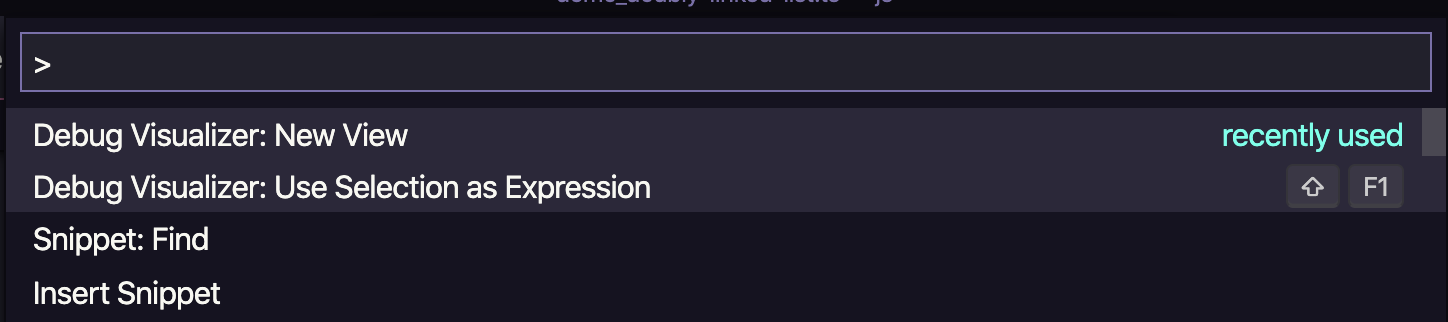You should now be able to enter `visualize()` into the prompt and see a plot visualization in your editor for the doubly linked list: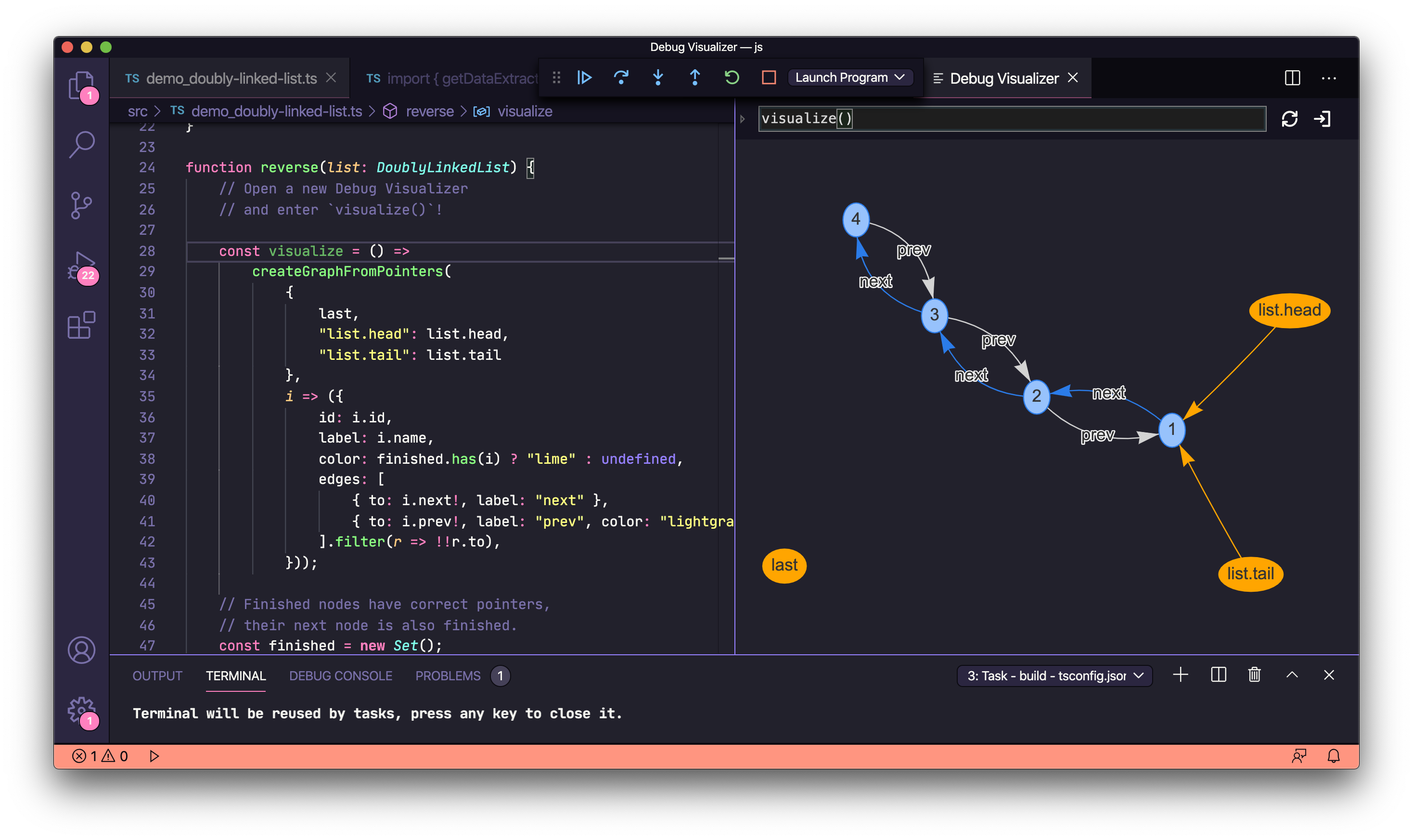Before trying the extension on your own examples, note that expressions you wish to visualize must both evaluate to a JSON Object string and match the schema of one of the extension's supported visualizers (outside of simple data types, like arrays). In the examples below, note the `"kind": { "graph": true }`, `"kind": { "plotly": true }` etc. entries which are a part of this schema.

Below you can find some of the code snippets I used when testing out the extension.

### Arrays

``````const range = [1, 2, 33, 31, 1, 2, 63, 943, 346, 24, 123, 6, 32];
``````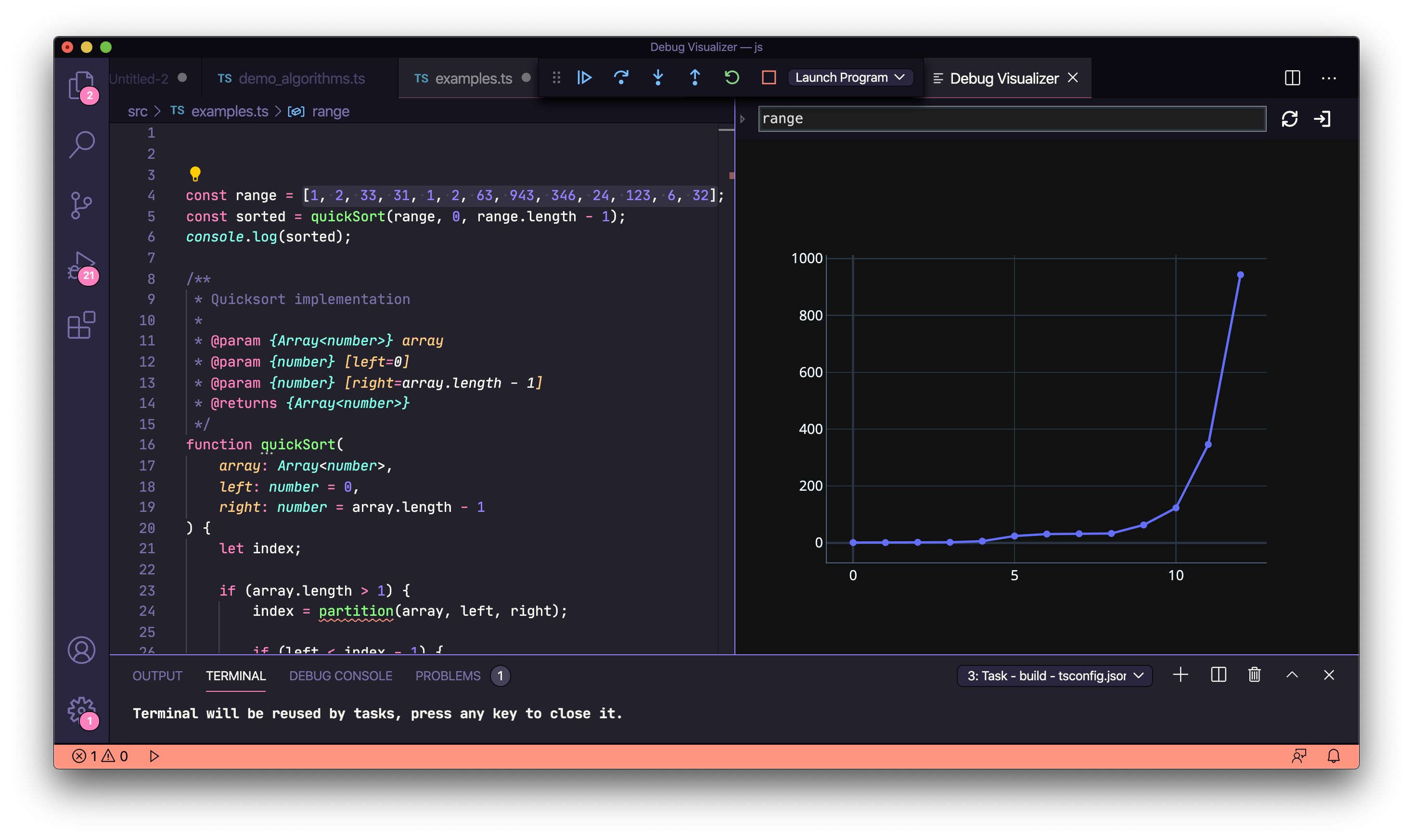### Dynamic data from a REST API

Data that is visualized doesn't need to be static. As demonstrated in the `fetch()` example below, it can also come from a REST API.

``````const fetch = require('node-fetch');

fetch('https://jsonplaceholder.typicode.com/users')
.then((response) => response.json())
.then((json) => {
debugger;
});
``````

### Graphs

``````const example1 = {
"kind": { "graph": true },
"nodes": [
{ "id": "1", "label": "1" },
{ "id": "2", "label": "2", "color": "orange" },
{ "id": "3", "label": "3" }
],
"edges": [
{ "from": "1", "to": "2", "color": "red" },
{ "from": "1", "to": "3" }
]
};
``````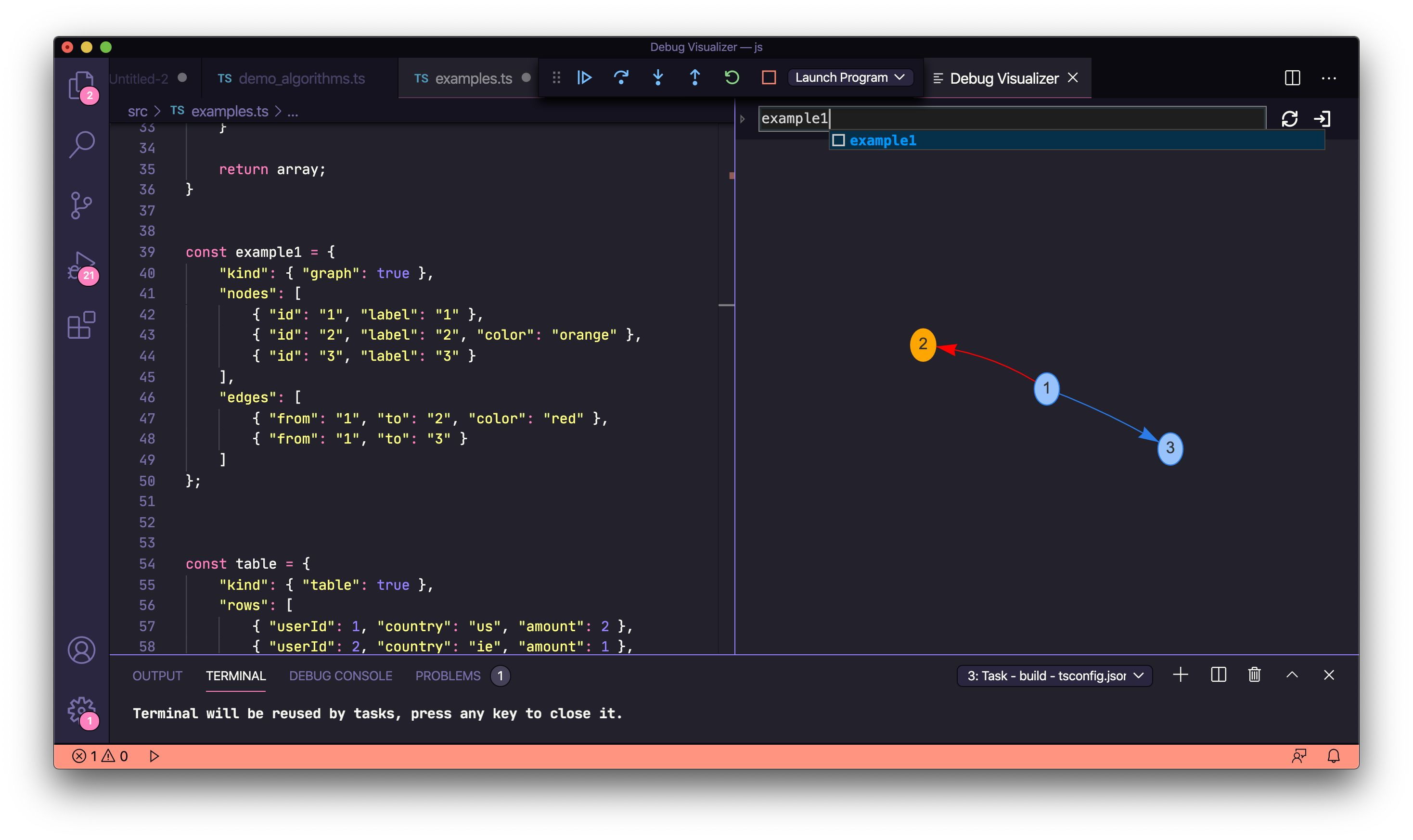### Tables

``````const table = {
"kind": { "table": true },
"rows": [
{ "userId": 1, "country": "us", "amount": 2 },
{ "userId": 2, "country": "ie", "amount": 1 },
{ "userId": 2, "country": "de", "amount": 1 },
{ "userId": 1, "country": "us" }
]
};
``````### Plotly graphs

`"kind": { "plotly": true }` allows you to leverage the Plotly graphing and data-science library for visualizations.

``````const example2 = {
"kind": {
"plotly": true
},
"data": [{
"y": [67, 58, 62, 65, 72],
"name": "Production"
},
{
"y": [69, 55, 67, 68, 73],
"name": "Staging"
}
]
};
``````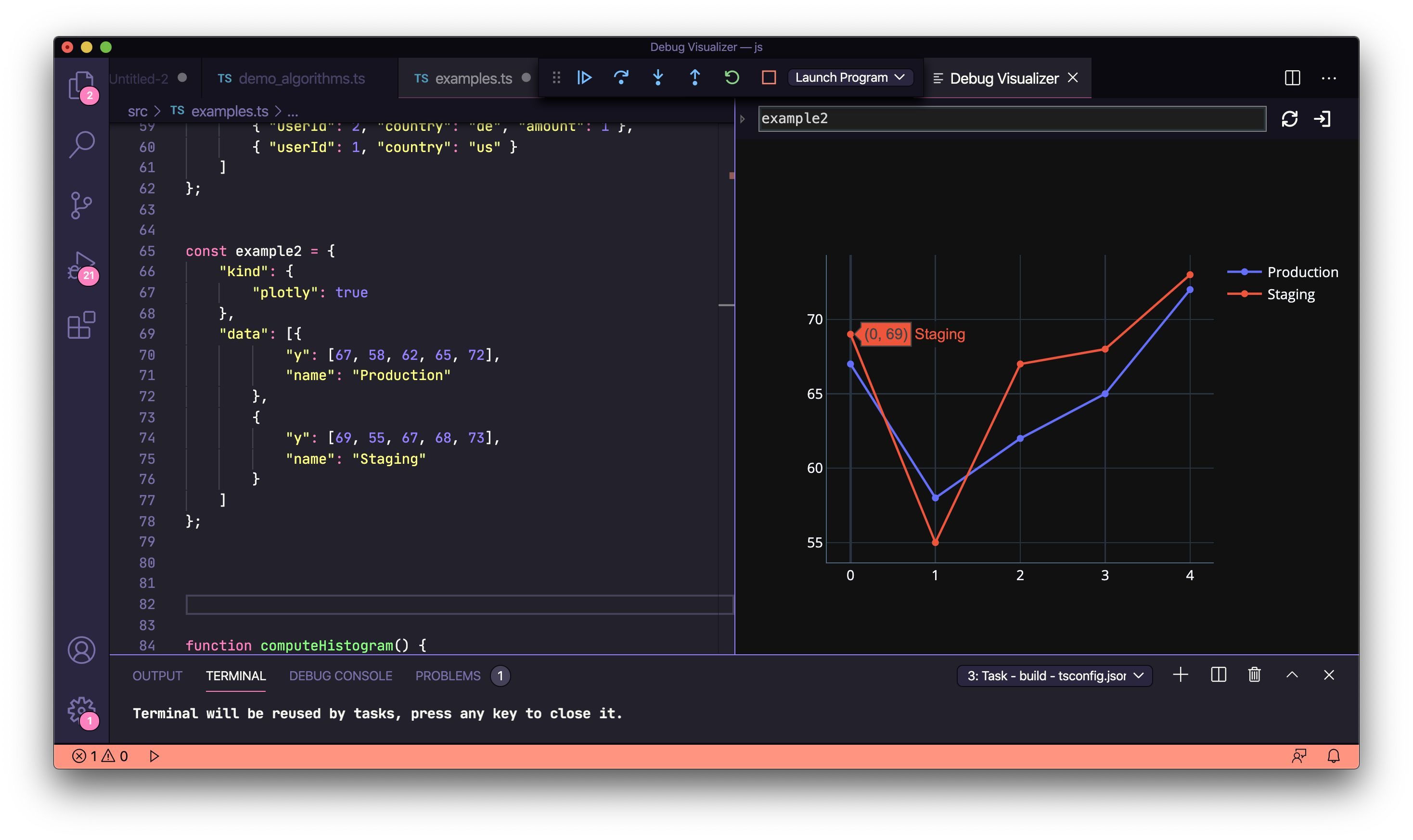### Plotly histograms

``````function computeHistogram() {
const x1 = [];
const x2 = [];
const y1 = [];
const y2 = [];
let k = 0;
for (let i = 1; i < 500; i++) {
k = Math.random();
x1.push(k * 5);
x2.push(k * 10);
y1.push(k);
y2.push(k * 2);
}
const trace1 = {
x: x1,
y: y1,
name: 'control',
autobinx: false,
histnorm: "count",
marker: {
color: "rgba(255, 100, 102, 0.7)",
line: {
color: "rgba(255, 100, 102, 1)",
width: 1
}
},
opacity: 0.5,
type: "histogram",
xbins: {
end: 2.8,
size: 0.06,
start: .5
}
};
const trace2 = {
x: x2,
y: y2,
autobinx: false,
marker: {
color: "rgba(100, 200, 102, 0.7)",
line: {
color: "rgba(100, 200, 102, 1)",
width: 1
}
},
name: "experimental",
opacity: 0.75,
type: "histogram",
xbins: {
end: 4,
size: 0.06,
start: -3.2

}
};
return {
"kind": {
"plotly": true
},
"data": [trace1, trace2],
"layout": {
bargap: 0.05,
bargroupgap: 0.2,
barmode: "overlay",
title: "Sampled Results",
xaxis: {
title: "Value"
},
yaxis: {
title: "Count"
}
}
};
}
let example3 = computeHistogram();
``````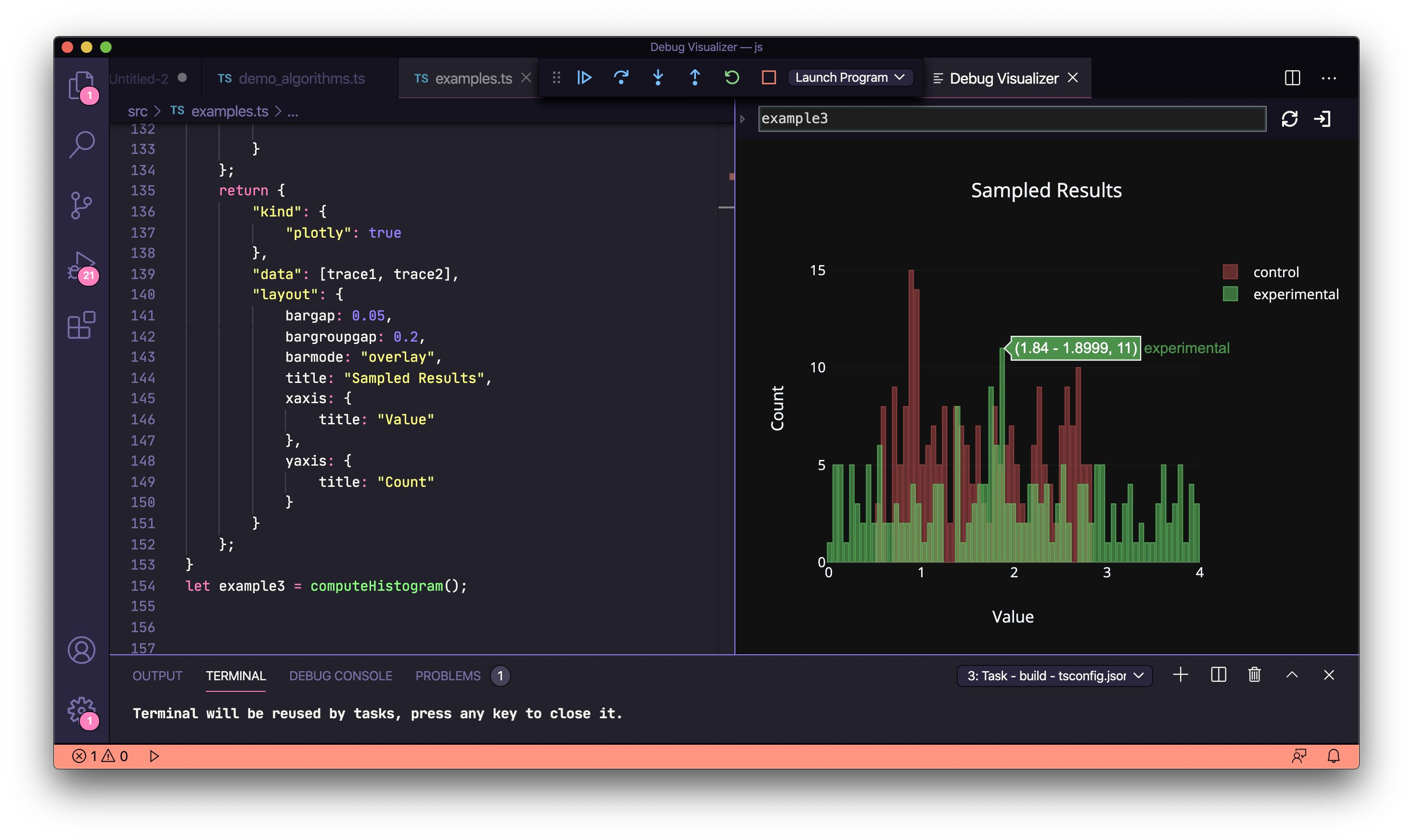### Plotly 3D-mesh graphs

``````// Generate random data
let a = [];
let b = [];
let c = [];
let a_ = 0;
let b_ = 0;
let c_ = 0;
for (let i = 0; i < 50; i++) {
a_ = Math.random();
a.push(a_);

b_ = Math.random();
b.push(b_);

c_ = Math.random();
c.push(c_);
}
// Plot the mesh
const example4 = {
"kind": {
"plotly": true
},
"data": [{
opacity: 0.8,
color: 'rgb(300,100,200)',
type: 'mesh3d',
x: a,
y: b,
z: c,
}]
};
``````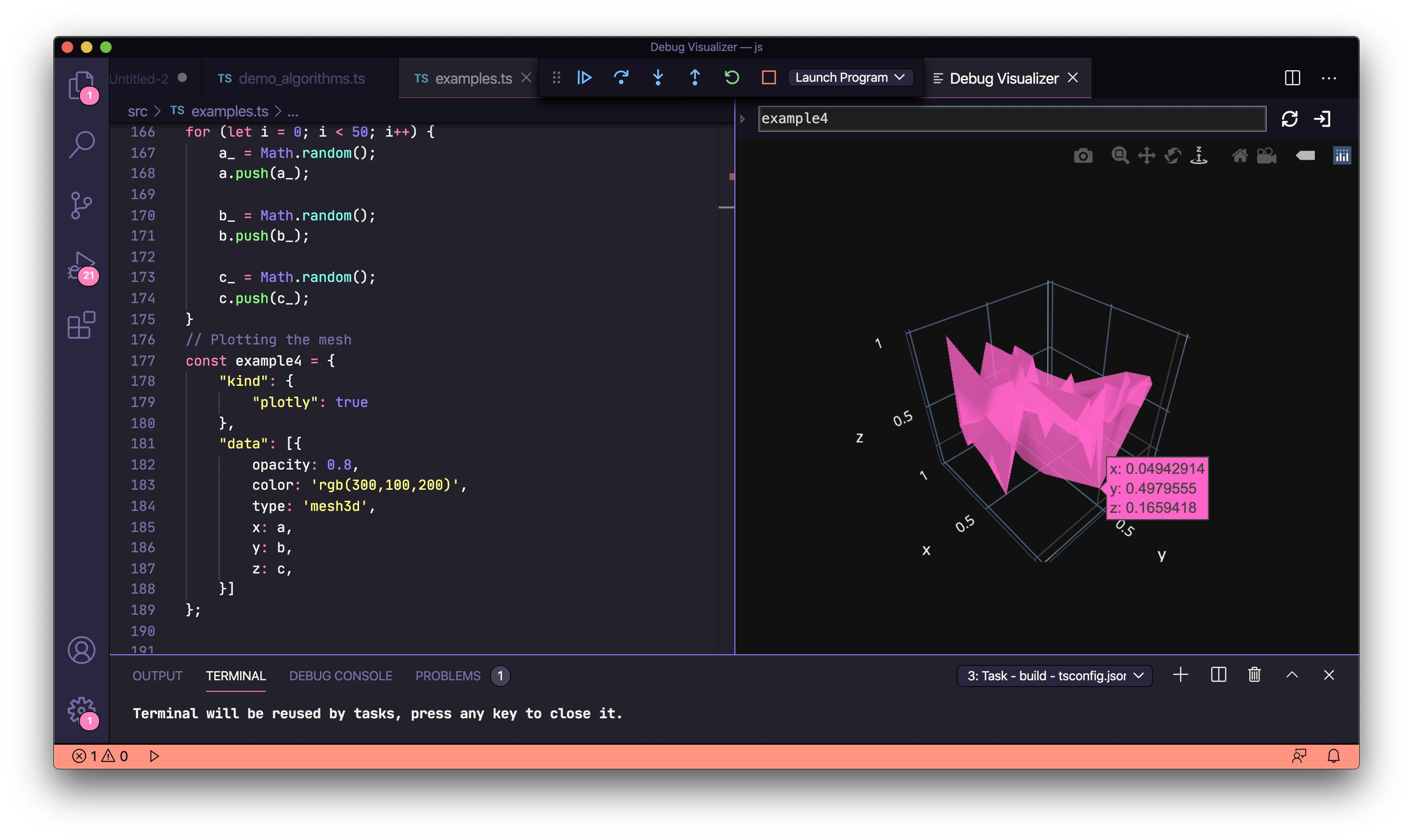### Plotly pie-charts

``````const example5 = {
"kind": {
"plotly": true
},
"data": {
values: [19, 26, 55],
labels: ['Work', 'Gym', 'Rest'],
type: 'pie'
},
"layout": {
height: 400,
width: 400
}
};
``````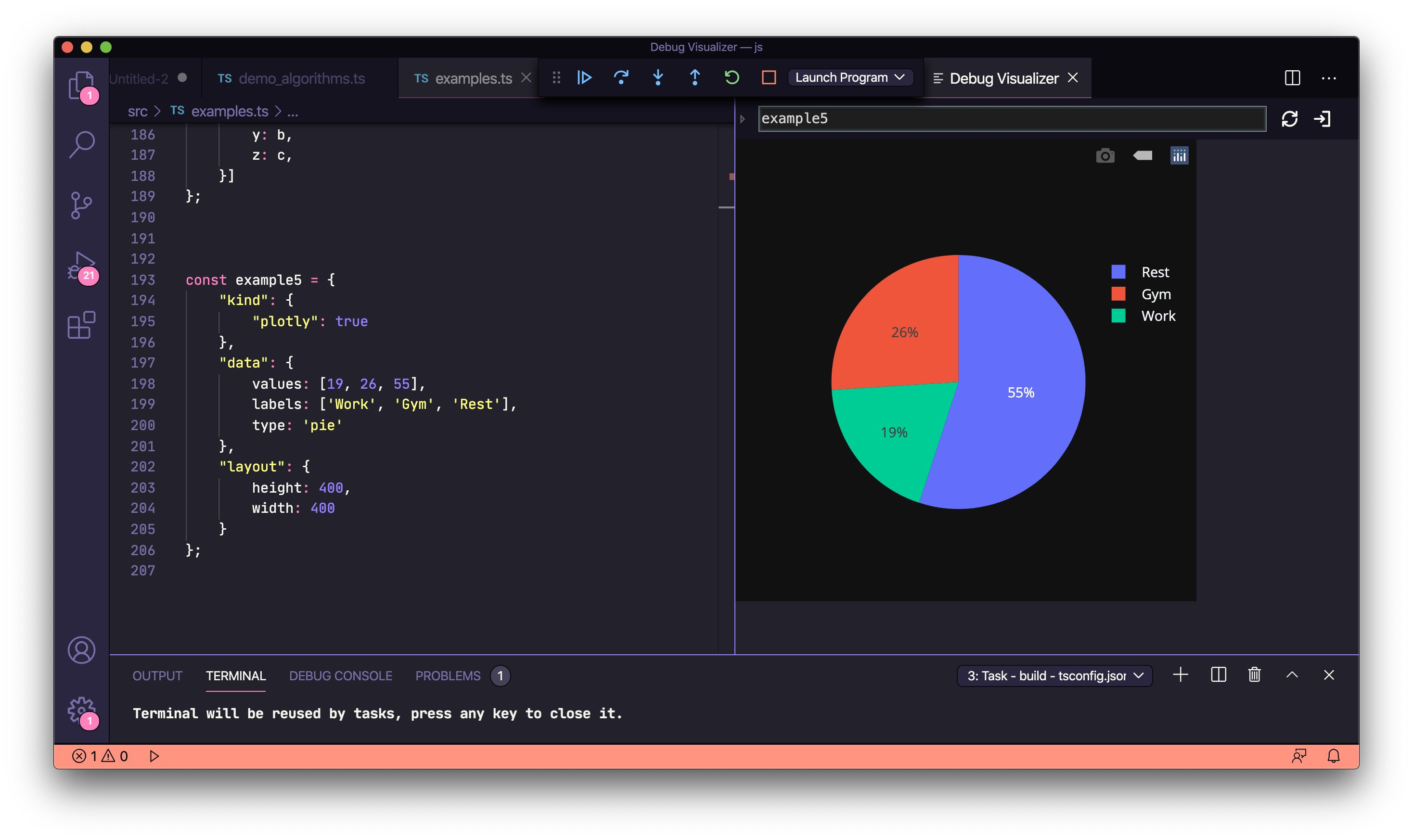Thanks to Henning Dieterichs for creating the extension. If you found this helpful, you may also be interested to try out the draw.io VSCode extension he also wrote.